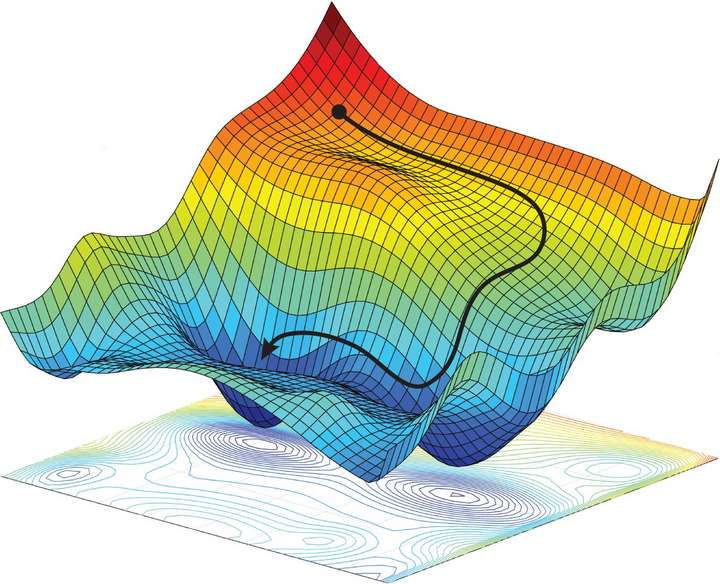top of page
Search

# Vanilla SGD, Mini Batch SGD, Mini Batch with Momentum, Mini Batch with Adam Optimization Techniquesa) Use the features extracted from the last fully-connected layer and train a multiclass SVM classifier on STL-10 dataset. Report the following

• Accuracy, Confusion Matrix on test data.

• ROC curve (assuming the chosen class as positive class and remaining classes as negative)

b) Fine-tune the ResNet 50 model (you may choose what layers to fine-tune) for the STL-10 dataset, and evaluate the classification performance on the test set before and after fine-tuning with respect to the following metrics,

• Class wise Accuracy

• Report Confusion Matrix.

[Code for accuracy, ROC, Confusion Matrix should be done from scratch, SVM - you may use sklearn]

• Triplet Loss as the final classification loss function

• Cross-Entropy as the final classification loss function

• Center loss as the final classification loss function

Choose any evaluation metrics (at least 3) and compare the models in a, b and c, comment on which one is better and why?

3. Implement a three layer CNN network, for classification task for the Dogs vs. Cats dataset. [You can use the necessary libraries/modules]

a. Compare the accuracy on the test dataset (split into train and test [70:30]) for the following optimization techniques :

• Vanilla SGD ii. Mini Batch SGD

• Mini Batch with momentum

b. Compare the accuracy on the test dataset (split into train and test [70:30]) for the following optimization techniques:

• Vanilla SGD

• Mini Batch SGD

• Mini Batch with momentum

d. What are the advantages of shuffling and partitioning the mini batches?

e. Explain the choice of beta (β) and how changing it changes the update. Explain the gradient update using plots.

f. Are there any advantages of using Adam over the other optimization methods?

Hire Realcode4you.com expert to get help in advance deep learning projects. If you are looking the solution of above problem then contact us at below mail id:

realcode4you@gmail.com